# How to implement a neural network - gradient descent

This page is the first part of this introduction on how to implement a neural network from scratch with Python. This first part will illustrate the concept of gradient descent illustrated on a very simple linear regression model. The next parts that follow on eachother will introduce classificaton in part 2, hidden layers trained by backpropagation in part 3, and vectorization of the operations in part 4.

## Gradient descent for linear regression ¶

In what follows we will go into the details of gradient descent illustrated on a very simple network: a 1-input 1-output linear regression model that has the goal to predict the target value $t$ from the input value $x$. The network is defined as having an input $\mathbf{x}$ which gets transformed by the weight $w$ to generate the output $\mathbf{y}$ by the formula $\mathbf{y} = w \cdot \mathbf{x}$, and where $\mathbf{y}$ needs to approximate the targets $\mathbf{t}$ as good as possible as defined by a loss function. This network can be represented graphically as: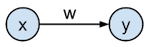In practice, we typically have multiple layers, non-linear activation functions, and a bias for each node. In this tutorial, we only have one layer with one weight parameter $w$, no activation function on the output, and no bias.

We will approximate the targets $\mathbf{t}$ with the outputs of the model $y$ by minimizing the squared error between target and output. This minimization will be performed by the gradient descent optimization algorithm which is typically used in training of neural networks.

In :

## Generating a toy dataset ¶

In this example, the targets $\mathbf{t}$ will be generated from a function $f$ and additive Gaussian noise sampled from $\mathcal{N}(0, 0.2)$, where $\mathcal{N}$ is the normal distribution with mean 0 and variance 0.2. $f$ is defined as $f(x) = 2 x$, with $\mathbf{x}$ the input samples, slope $2$ and intercept $0$. $\mathbf{t}$ is $f(\mathbf{x}) + \mathcal{N}(0, 0.2)$.

We will sample 20 input samples $\mathbf{x}$ from the uniform distribution between 0 and 1, and then generate the target output values $\mathbf{t}$ by the process described above. These resulting inputs $\mathbf{x}$ and targets $\mathbf{t}$ are plotted against each other in the figure below together with the original $f(x)$ line without the gaussian noise. Note that $\mathbf{x}$ is a vector of individual input samples $x_i$, and that $\mathbf{t}$ is a corresponding vector of target values $t_i$.

In :
In :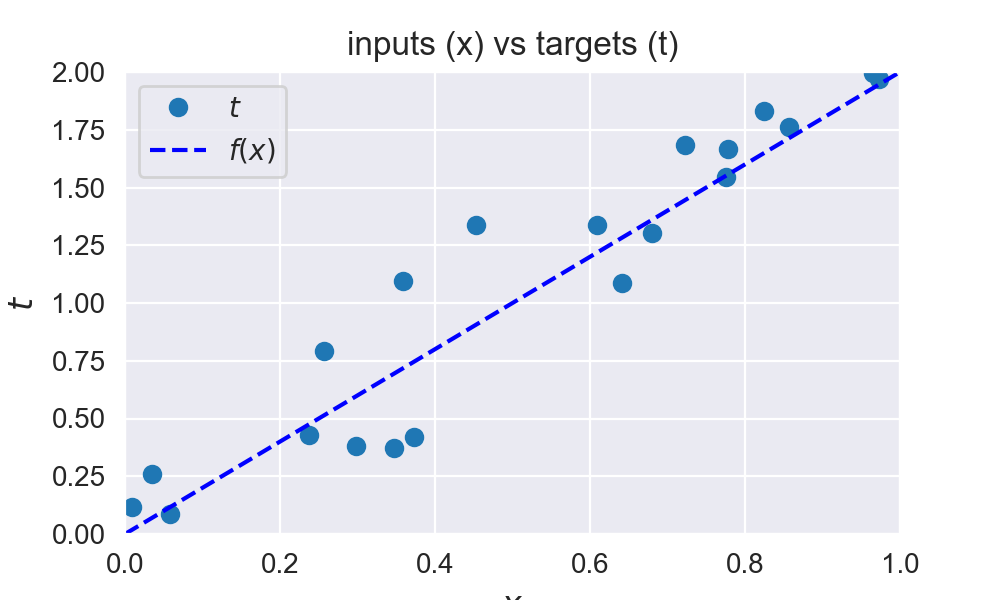## Define the loss function ¶

We will optimize the model $\mathbf{y} = \mathbf{x} * w$ by tuning parameter $w$ so that the mean squared error (MSE) along all samples is minimized. The function to minimizize is also known as the loss (or cost) function .

The mean squared error is defined as $\xi = \frac{1}{N} \sum_{i=1}^{N} \Vert t_i - y_i \Vert ^2$, with $N$ the number of samples in the training set. This corresponds to the average Euclidian distance between the output and corresponding targets. The optimization goal is thus: $\underset{w}{\text{argmin}} \frac{1}{N} \sum_{i=1}^{N} \Vert t_i - y_i \Vert^2$.
Notice that we take the mean of errors over all samples, which is known as batch training. We could also update the parameters based upon one sample at a time, which is known as online training.

This loss function for variable $w$ is plotted in the figure below. The value $w=2$ is at the minimum of the loss function (bottom of the parabola), this value is the same value as the slope we choose for $f(x)$. Notice that this function is convex and that there is only one minimum: the global minimum. While every squared error loss function for linear regression is convex, this is not the case for other models and other loss functions.

The neural network model is implemented in the  nn(x, w)  function, and the loss function is implemented in the  loss(y, t)  function.

In :
def nn(x, w):
"""Output function y = x * w"""
return x * w

def loss(y, t):
"""MSE loss function"""
return np.mean((t - y)**2)

In :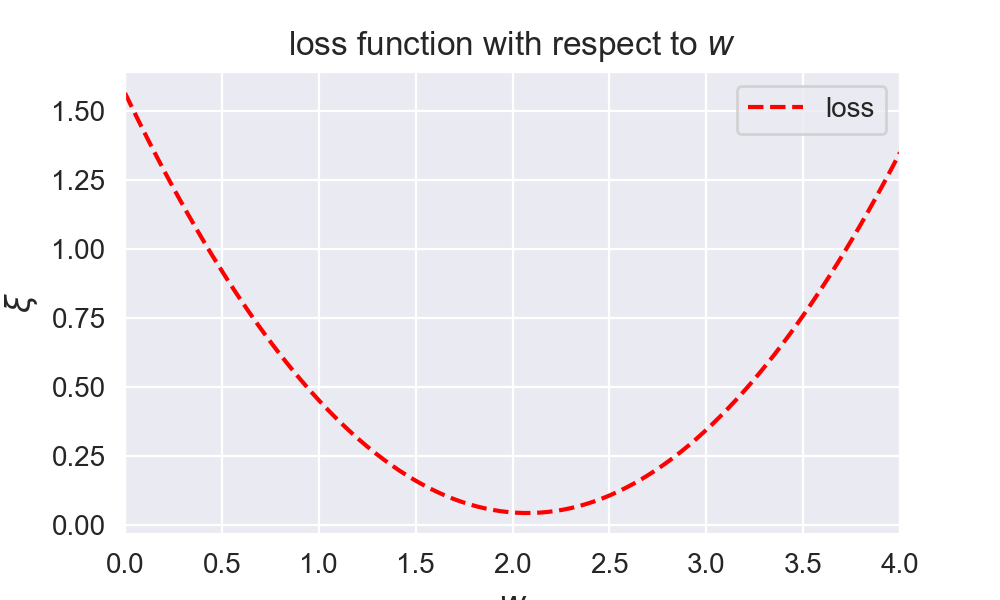## Minimizing the loss function ¶

For a simple loss function like in this example, you can see easily what the optimal weight should be. However, in practice the error surface can be quite complex with many hills and valleys, or have a high dimensionality (each parameter adds a new dimension). This is why we use optimization techniques like gradient descent to find the minimum of the error function.

One optimization algorithm commonly used to train neural networks is the gradient descent algorithm. The gradient descent algorithm works by taking the gradient ( derivative ) of the loss function $\xi$ with respect to the parameters at a specific position on this loss function, and updates the parameters in the direction of the negative gradient (down along the loss function). The parameter $w$ is iteratively updated by taking steps proportional to the negative of the gradient: $$w(k+1) = w(k) - \Delta w(k)$$

With $w(k)$ the value of $w$ at iteration $k$ during the gradient descent.
$\Delta w$ is defined as: $$\Delta w = \mu \frac{\partial \xi}{\partial w}$$

With $\mu$ the learning rate, which is how big of a step you take along the gradient, and ${\partial \xi}/{\partial w}$ the gradient of the loss function $\xi$ with respect to the weight $w$. For each sample $i$ this gradient can be splitted according to the chain rule into:

$$\frac{\partial \xi_i}{\partial w} = \frac{\partial \xi_i}{\partial y_i} \frac{\partial y_i}{\partial w}$$

Where $\xi_i$ is the squared error loss, so the ${\partial \xi_i}/{\partial y_i}$ term can be written as:

$$\frac{\partial \xi_i}{\partial y_i} = \frac{\partial (t_i - y_i)^2}{\partial y_i} = - 2 (t_i - y_i) = 2 (y_i - t_i)$$

And since $y_i = x_i \cdot w$ we can write ${\partial y_i}/{\partial w}$ as:

$$\frac{\partial y_i}{\partial w} = \frac{\partial (x_i \cdot w)}{\partial w} = x_i$$

So the full update function $\Delta w$ for sample $i$ will become:

$$\Delta w = \mu \cdot \frac{\partial \xi_i}{\partial w} = \mu \cdot 2 x_i (y_i - t_i)$$

In the batch processing, we just add up all the gradients for each sample:

$$\Delta w = \mu * 2 * \frac{1}{N} \sum_{i=1}^{N} x_i (y_i - t_i)$$

The gradient descent algorithm is typically initialised by starting with random initial parameters. After initiating these parameters we can start updating these parameters with $\Delta w$ until convergence. The learning rate needs to be tuned separately as a hyperparameter for each neural network.

The gradient ${\partial \xi}/{\partial w}$ is implemented by the  gradient(w, x, t)  function. $\Delta w$ is computed by the  delta_w(w_k, x, t, learning_rate)  . The loop below performs 4 iterations of gradient descent while printing out the parameter value and current loss.

In :
def gradient(w, x, t):
"""Gradient function. (Remember that y = nn(x, w) = x * w)"""
return 2 * x * (nn(x, w) - t)

def delta_w(w_k, x, t, learning_rate):
"""Update function delta w"""
return learning_rate * np.mean(gradient(w_k, x, t))

# Initial weight parameter
w = np.random.rand()
# Set the learning rate
learning_rate = 0.9

# Perform the gradient descent updates, and print the weights and loss:
w_loss = [(w, loss(nn(x, w), t))] # Keep track of weight and loss values
for i in range(nb_of_iterations):
dw = delta_w(w, x, t, learning_rate)  # Delta w update
w = w - dw  # Update the current weight parameter
w_loss.append((w, loss(nn(x, w), t)))  # Save weight and loss

# Print the final w, and loss
for i in range(0, len(w_loss)):
print(f'w({i}): {w_loss[i]:.4f} \t loss: {w_loss[i]:.4f}')

w(0): 0.1380 	 loss: 1.3710
w(1): 1.3722 	 loss: 0.2174
w(2): 1.8205 	 loss: 0.0651
w(3): 1.9834 	 loss: 0.0451
w(4): 2.0426 	 loss: 0.0424


Notice in the previous outcome that the gradient descent algorithm quickly converges towards the target value around $2.0$. Let's try to plot these iterations of the gradient descent algorithm to visualize it.

In :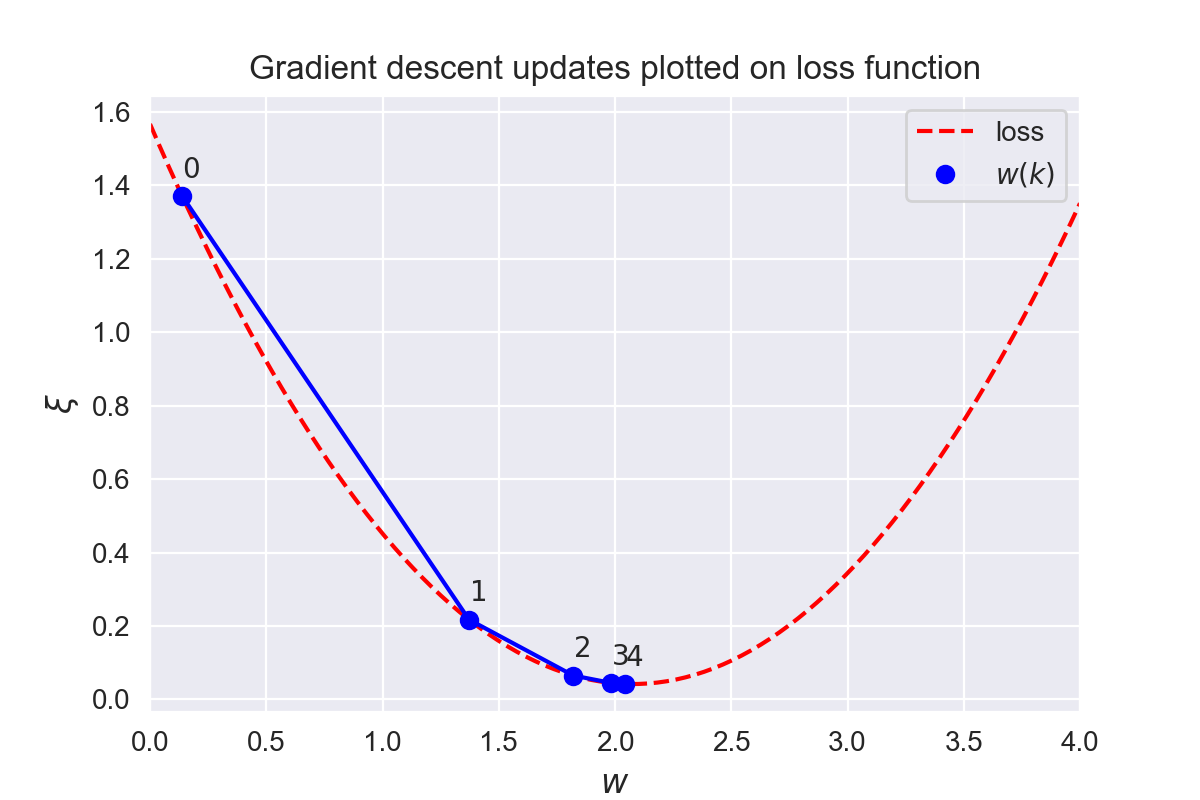The previous figure shows the gradient descent updates of the weight parameters for 4 iterations. The blue dots represent the weight parameter values $w(k)$ at iteration $k$. Notice how the update differs from the position of the weight and the gradient at that point. The first update takes a much larger step than the second update because the gradient at $w(0)$ is much larger than the gradient at $w(1)$.

The regression line fitted by gradient descent with 10 iterations is shown in the figure below. The resulting line fitted with gradient descent (full red) lies close to the original line that generated the samples(dashed blue). Notice that both lines go through point $(0,0)$, this is because we didn't have a bias term, which represents the intercept, the intercept at $x=0$ is thus $t=0$.

In :
w = np.random.rand()
for i in range(nb_of_iterations):
dw = delta_w(w, x, t, learning_rate)  # get the delta w update
w = w - dw  # update the current weight parameter

In :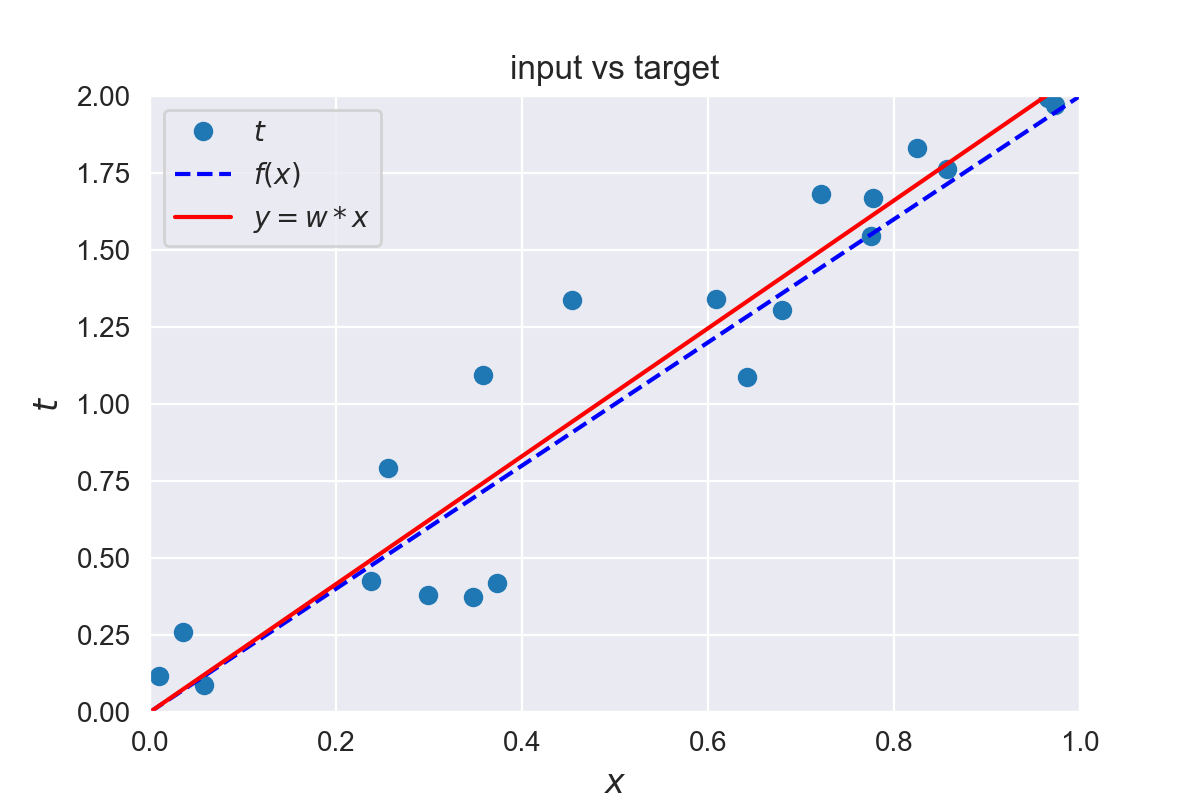In :
Python: 3.7.0
numpy: 1.15.2
matplotlib: 3.0.0
seaborn: 0.9.0


This was the first part of a 4-part tutorial on how to implement neural networks from scratch in Python:

The tutorials are generated from Python 3 Jupyter notebook files, which will be linked to at the end of each chapter so that you can adapt and run the examples yourself. The neural networks themselves are implemented using the Python NumPy library which offers efficient implementations of linear algebra functions such as vector and matrix multiplications. Illustrative plots are generated using Matplotlib and Seaborn . Libraries were installed via the Anaconda Python distribution.

Note that the code input cells in this blog can be collapsed or expanded by clicking on the button in the top right of each cell.

This post at peterroelants.github.io is generated from an IPython notebook file. Link to the full IPython notebook file